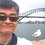# Proof of an integral involving even and odd functions

This note provides a proof of the following identity

$\int_{-a}^a \frac {f(x)}{1+c^{g(x)}} \ dx = \int_0^a f(x)\ dx$

where $f(x)$ is an even function, $g(x)$, an odd function, and $a$ and $c$ are constants.

Proof:

\begin{aligned} \int_{-a}^a \frac {f(x)}{1+c^{g(x)}} \ dx & = {\color{#3D99F6} \int_{-a}^0 \frac {f(x)}{1+c^{g(x)}} \ dx} + \int_0^a \frac {f(x)}{1+c^{g(x)}} \ dx & \small \color{#3D99F6} \text{Let }u=-x \implies dx = -du \\ & = {\color{#3D99F6} - \int_a^0 \frac {f(-u)}{1+c^{g(-u)}} \ du} + \int_0^a \frac {f(x)}{1+c^{g(x)}} \ dx & \small \color{#3D99F6} \text{Since for even function }f(-u) = f(u) \\ & = {\color{#3D99F6} \int_0^a \frac {f(u)}{1+c^{-g(u)}} \ du} + \int_0^a \frac {f(x)}{1+c^{g(x)}} \ dx & \small \color{#3D99F6} \text{ and odd function }g(-u) = - g(u) \\ & = {\color{#3D99F6} \int_0^a \frac {c^{g(u)} f(u)}{1+c^{g(u)}} \ du} + \int_0^a \frac {f(x)}{1+c^{g(x)}} \ dx & \small \color{#3D99F6} \text{Replace }u \text{ with }x \\ & = \int_0^a \frac {\left(1+c^{g(x)}\right) f(x)}{1+c^{g(x)}} \ dx \\ & = \int_0^a f(x) \ dx \quad \blacksquare \end{aligned}Note by Chew-Seong Cheong
1 year, 11 months ago

This discussion board is a place to discuss our Daily Challenges and the math and science related to those challenges. Explanations are more than just a solution — they should explain the steps and thinking strategies that you used to obtain the solution. Comments should further the discussion of math and science.

When posting on Brilliant:

• Use the emojis to react to an explanation, whether you're congratulating a job well done , or just really confused .
• Ask specific questions about the challenge or the steps in somebody's explanation. Well-posed questions can add a lot to the discussion, but posting "I don't understand!" doesn't help anyone.
• Try to contribute something new to the discussion, whether it is an extension, generalization or other idea related to the challenge.

MarkdownAppears as
*italics* or _italics_ italics
**bold** or __bold__ bold
- bulleted- list
• bulleted
• list
1. numbered2. list
1. numbered
2. list
Note: you must add a full line of space before and after lists for them to show up correctly
paragraph 1paragraph 2

paragraph 1

paragraph 2

[example link](https://brilliant.org)example link
> This is a quote
This is a quote
    # I indented these lines
# 4 spaces, and now they show
# up as a code block.

print "hello world"
# I indented these lines
# 4 spaces, and now they show
# up as a code block.

print "hello world"
MathAppears as
Remember to wrap math in $$ ... $$ or $ ... $ to ensure proper formatting.
2 \times 3 $2 \times 3$
2^{34} $2^{34}$
a_{i-1} $a_{i-1}$
\frac{2}{3} $\frac{2}{3}$
\sqrt{2} $\sqrt{2}$
\sum_{i=1}^3 $\sum_{i=1}^3$
\sin \theta $\sin \theta$
\boxed{123} $\boxed{123}$

Sort by:

so g can be any function that is odd

- 1 year, 8 months ago

Yes, I use a general odd function $g(x)$ and no other condition.

- 1 year, 8 months ago

did you do this by your self

- 1 year, 8 months ago

I didn't discover it. I have seen two examples of its use and I put it into this note so that I can use it in my solutions in the future.

- 1 year, 8 months ago